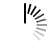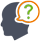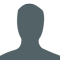Diwali Bonanza | Get 30-Days Free Fun-Filled Learning | Subscribe Now1800-833-6464 Buy Activation Why Tutorix Features Login

#DoubtTushar

In figure below, if $AB\ ∥\ CD$. If $OA\ =\ 3x\ –\ 19$, $OB\ =\ x\ –\ 4$, $OC\ =\ x\ -\ 3$ and $OD\ =\ 4$, find $x$.Solutions :Akhilesh

Given:

In the given figure $AB\ ∠¥\ CD$.

”Š$OA\ =\ 3x\ –\ 19$, $OB\ =\ x\ –\ 4$, $OC\ =\ x\ -\ 3$ and $OD\ =\ 4$.

To do:

We have to find the value of $x$.

Solution:

We know that,

Diagonals of a Trapezium divide each other proportionally.

Therefore,

$\begin{array}{l} \frac{AO}{CO} =\frac{BO}{DO}\\ \\ \frac{3x-19}{x-3} =\frac{x-4}{4}\\ \\ 4( 3x-19) =( x-4)( x-3)\\ \\ 4( 3x-19) =x( x-3) -4( x-3)\\ \\ 12x -76=x^{2} -3x-4x+12\\ \\ x^{2} +( -3-4-12) x+( 12+76) =0\\ \\ x^{2} -19x+88=0\\ \\ x^{2} -11x-8x+88=0\\ \\ x( x-11) -8( x-11) =0\\ \\ ( x-11)( x-8) =0\\ \\ x-11=0\ \ \ or\ \ x-8=0\\ \\ x=11\ \ \ \ or\ \ \ x=8 \end{array}$

Hence, the value of $x$ is $8$ or $11$.

0

﻿